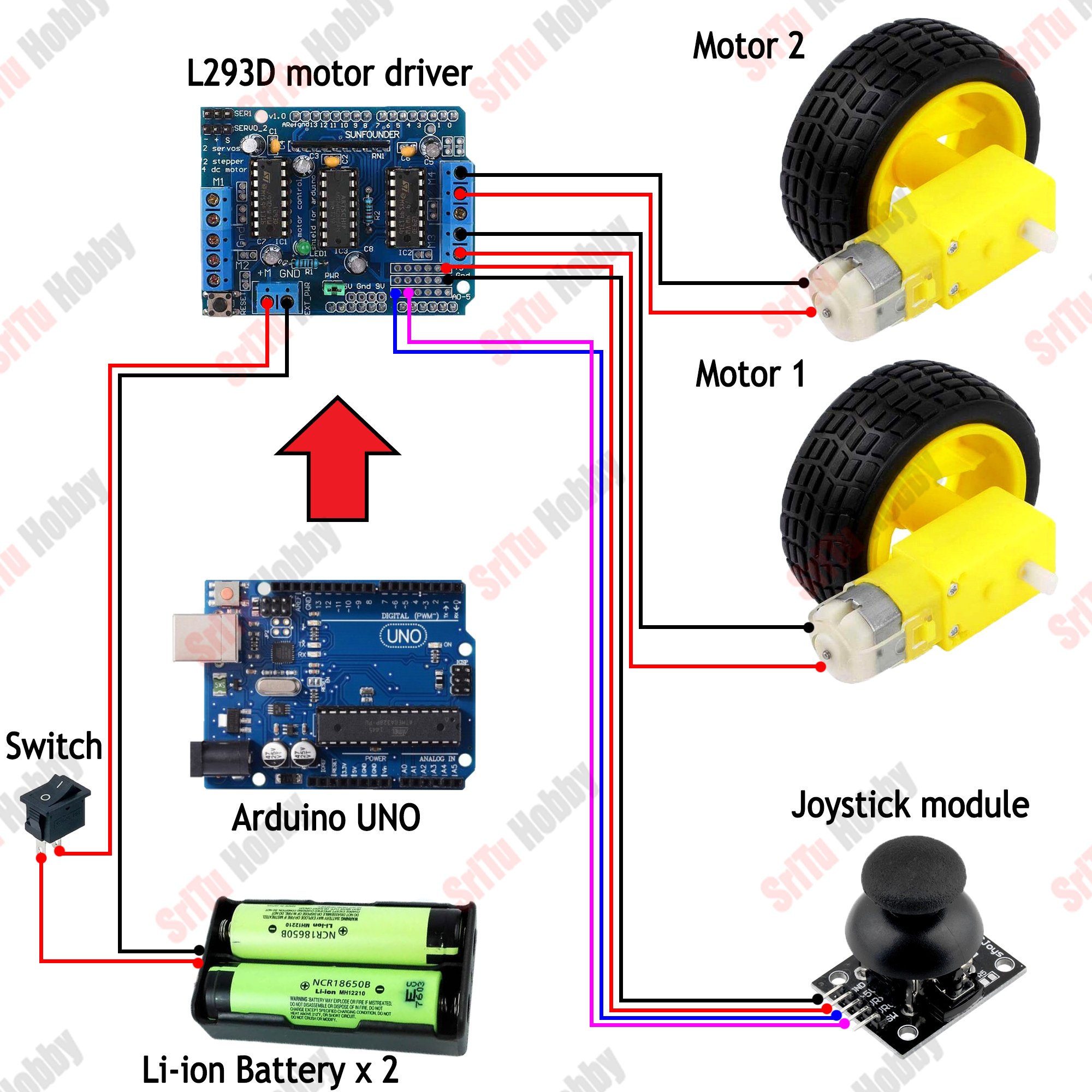# How to make a DIY joystick control car with Arduino | Step by step instructions

Hello, welcome back to another tutorial from the SriTu Hobby. This tutorial includes how to make a joystick module control car with Arduino. Also, this project mainly used the Arduino UNO board and L293D motor driver shield. I hope you will have the knowledge to try out this design on your own by the end of this tutorial. Also. we have provided step-by-step instructions on how you can create this project in-house at a very low cost.

### The process of this project

When the project is powered on, the Arduino board and motor driver board are activated and the car is activated. Remember to give a potential of 7.4 to 12 in particular. Then, when the joystick module is moved, the analog values are received by the Arduino board. Also, when the joystick module is moving forward, the car moves forward, when moving backward the car moves backward, when moving right the car moves right, when moving left the car moves left. All of this is set up in the program.

OK, let’s do this project step by step. The required components are given below.

### Step 1

Firstly, identify these components.

### Step 2

Secondly, cut the foam board as follows.

### Step 3

Thirdly, let’s cut the car chassis. Cut it as follows.

### Step 4

Now, glue the two gear motors to this chassis.

### Step 5

Then attach the rotating wheel as follows.

### Step 6

Now, attach the motor drive shield to the Arduino UNO board. Then, glue this to the car chassis.

### Step 7

Afterward, connect the gear motors to the motor driver shield. To do this, use the circuit diagram below.### Step 8

Now, glue the battery holder as follows. After, connect it to the motor driver shield.

### Step 9

Then, connect the joystick module to the motor driver shield. To do this, use the circuit diagram above.

### Step 10

After, connect this car to the computer. Ok, let’s creates the program for this project. It is as follows.

``````/*joystic car control project.
* https://srituhobby.com
*/

#include <AFMotor.h>
#define x A0
#define y A1
#define Speed 180

AF_DCMotor motor1(3);
AF_DCMotor motor2(4);

void setup() {
Serial.begin(9600);
motor1.setSpeed(Speed);
motor2.setSpeed(Speed);
}

void loop() {

Serial.print(X);
Serial.print("\t");
Serial.println(Y);

if (X >= 800) {
motor1.run(BACKWARD);
motor2.run(BACKWARD);
} else if (X <= 200) {
motor1.run(FORWARD);
motor2.run(FORWARD);
} else if (Y >= 800) {
motor1.run(FORWARD);
motor2.run(BACKWARD);
} else if (Y <= 200) {
motor1.run(BACKWARD);
motor2.run(FORWARD);
} else {
motor1.run(RELEASE);
motor2.run(RELEASE);
}
}``````

#### Code explanation

Firstly, the AF motor library is included.
#include <AFMotor.h>

Secondly, the joystick module input pins and car speed are defined.
#define x A0
#define y A1
#define Speed 180

Third, objects are created for the terminals we use. Example:- terminal 3 as motor1, terminal 4 as motor2.
AF_DCMotor motor1(3);
AF_DCMotor motor2(4);

In the setup function, the serial monitor starts. Also, the motor speed is set.
void setup() {
Serial.begin(9600);
motor1.setSpeed(Speed);
motor2.setSpeed(Speed);
}

In the loop function, the analog values are obtained through the joystick module and inserted into the integer variables. Also, these values are printed on the serial monitor. Then, these values are checked using the IF condition. If the X value is greater than and equal to 800, the car moves forward. If the X value is less than and equal to 200, the car moves backward. If the Y value is greater than and equal 800, the car turns left. If the Y value is less than and equal 200, the car turns right. Otherwise, the car will stop.
void loop() {

Serial.print(X);
Serial.print(“\t”);
Serial.println(Y);

if (X >= 800) {
motor1.run(BACKWARD);
motor2.run(BACKWARD);
} else if (X <= 200) {
motor1.run(FORWARD);
motor2.run(FORWARD);
} else if (Y >= 800) {
motor1.run(FORWARD);
motor2.run(BACKWARD);
} else if (Y <= 200) {
motor1.run(BACKWARD);
motor2.run(FORWARD);
} else {
motor1.run(RELEASE);
motor2.run(RELEASE);
}
}

### Step 11

OK, now select board and port. Then, upload this code to the Arduino board.

### Step 12

Lastly, put the batteries to the battery holder and switch ON.

OK, enjoy this project. The full video guide is given below. So, we will meet in the next tutorial.

### 4 thoughts on “How to make a DIY joystick control car with Arduino | Step by step instructions”

1. Good the program gives errors when uploading it Arduino in the reverse gear change section so it does not work given I could allude to the problem
thanks from Spain
my email is [email protected]